# 关键点回顾¶

## 直接位置预测¶

YOLOv2借鉴RPN网络使用Anchor boxes来预测边界框相对于先验框的offsets。边界框的实际中心位置$(x,y)$需要利用预测的坐标偏移值$(t_x,t_y)$，先验框的尺度$(w_a,h_a)$以及中心坐标$(x_a,y_a)$来计算，这里的$x_a$$y_a$也即是特征图每个位置的中心点：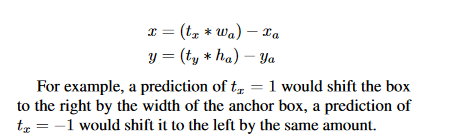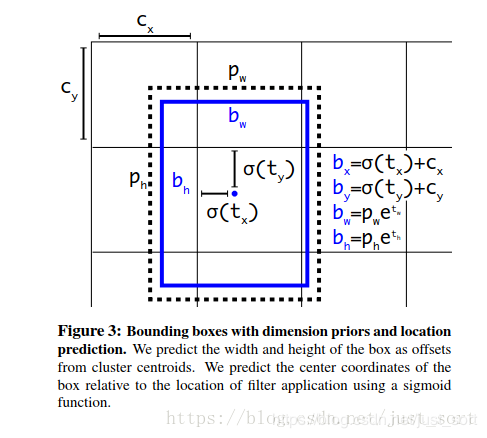$b_x=(\sigma(t_x)+c_x)/W$ $b_y=(\sigma(t_y)+c_y)/H$ $b_w=p_we^{t_w}/W$ $b_h=p_he^{t_h}/H$

## 细粒度特征¶

YOLOv2提取Darknet-19最后一个max pool层的输入，得到26x26x512的特征图。经过1x1x64的卷积以降低特征图的维度，得到26x26x64的特征图，然后经过pass through层的处理变成13x13x256的特征图（抽取原特征图每个2x2的局部区域组成新的channel，即原特征图大小降低4倍，channel增加4倍），再与13x13x1024大小的特征图连接，变成13x13x1280的特征图，最后在这些特征图上做预测。使用Fine-Grained Features，YOLOv2的性能提升了1%。这个过程可以在下面的YOLOv2的结构图中看得很清楚：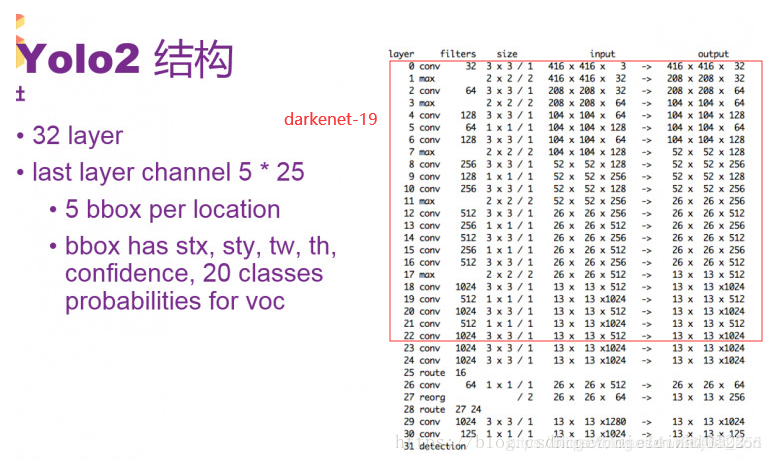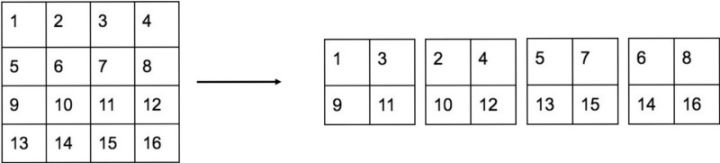# YOLOv2的模型结构¶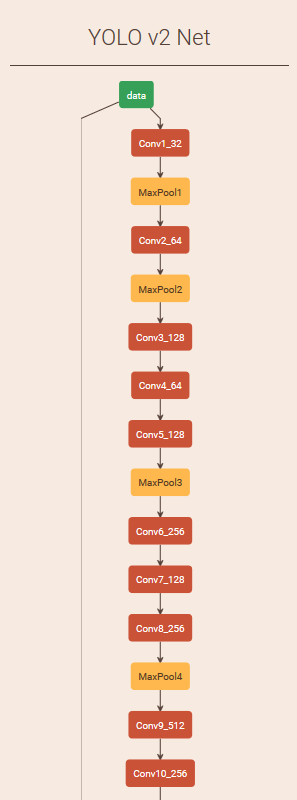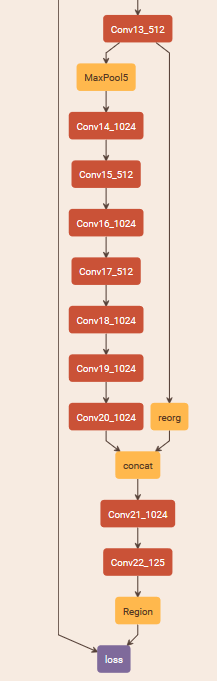# 损失函数¶

## 解释1¶

YOLOv2的损失函数和YOLOv1一样，对于训练集中的ground truth，中心落在哪个cell，那么该cell的5个Anchor box对应的边界框就负责预测它，具体由哪一个预测同样也是根据IOU计算后卡阈值来确定的，最后选IOU值最大的那个。这也是建立在每个Cell至多含有一个目标的情下，实际上也基本不会出现多余1个的情况。和ground truth匹配上的先验框负责计算坐标误差，置信度误差以及分类误差，而其它4个边界框只计算置信度误差。这个解释参考的YOLOv2实现是darkflow.源码地址为：https://github.com/thtrieu/darkflow

## 解释2¶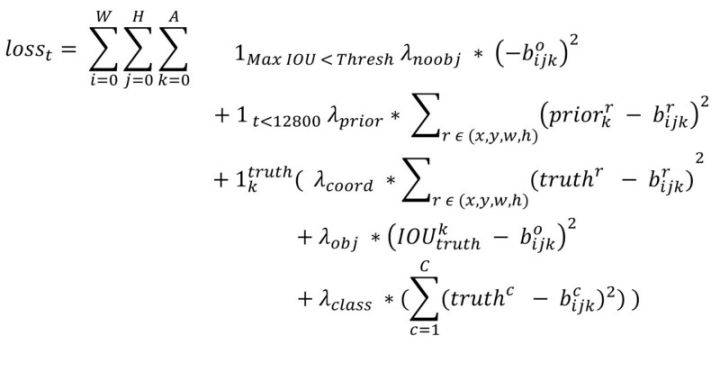• 第一部分：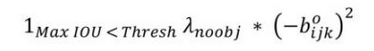第一项需要好好解释一下，这个loss是计算background的置信度误差，这也是YOLO系列算法的特色，但是用哪些预测框来预测背景呢？这里需要计算各个预测框和所有的ground truth之间的IOU值，并且取最大值记作MaxIOU，如果该值小于一定的阈值，YOLOv2论文取了0.6，那么这个预测框就标记为background，需要计算$\lambda_{noobj}$这么多倍的损失函数。为什么这个公式可以这样表达呢？因为我们有物体的话，那么$\lambda_{noobj}=0$，如果没有物体$\lambda_{noobj}=1$，我们把这个值带入到下面的公式就可以推出第一项啦！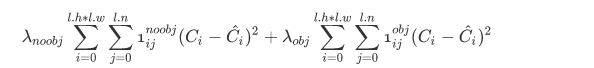• 第二部分：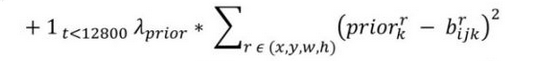• 第三部分：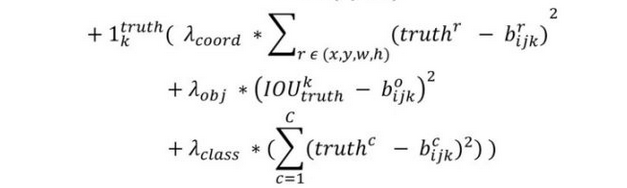3.1 坐标损失 这里的匹配原则是指对于某个特定的ground truth，首先要计算其中心点落在哪个cell上，然后计算这个cell的5个先验框和grond truth的IOU值，计算IOU值的时候不考虑坐标只考虑形状，所以先将Anchor boxes和ground truth的中心都偏移到同一位置，然后计算出对应的IOU值，IOU值最大的先验框和ground truth匹配，对应的预测框用来预测这个ground truth。

3.2 置信度损失 在计算obj置信度时， 增加了一项$\lambda_{obj}$权重系数，也被称为rescore参数，当其为1时，损失是预测框和ground truth的真实IOU值(darknet中采用了这种实现方式)。而对于没有和ground truth匹配的先验框，除去那些Max_IOU低于阈值的，其它就全部忽略。YOLOv2和SSD与RPN网络的处理方式有很大不同，因为它们可以将一个ground truth分配给多个先验框。

3.3 分类损失 这个和YOLOv1一致，没什么好说的了。

# 代码实现¶

def darknet(images, n_last_channels=425):
"""Darknet19 for YOLOv2"""
net = conv2d(images, 32, 3, 1, name="conv1")
net = maxpool(net, name="pool1")
net = conv2d(net, 64, 3, 1, name="conv2")
net = maxpool(net, name="pool2")
net = conv2d(net, 128, 3, 1, name="conv3_1")
net = conv2d(net, 64, 1, name="conv3_2")
net = conv2d(net, 128, 3, 1, name="conv3_3")
net = maxpool(net, name="pool3")
net = conv2d(net, 256, 3, 1, name="conv4_1")
net = conv2d(net, 128, 1, name="conv4_2")
net = conv2d(net, 256, 3, 1, name="conv4_3")
net = maxpool(net, name="pool4")
net = conv2d(net, 512, 3, 1, name="conv5_1")
net = conv2d(net, 256, 1, name="conv5_2")
net = conv2d(net, 512, 3, 1, name="conv5_3")
net = conv2d(net, 256, 1, name="conv5_4")
net = conv2d(net, 512, 3, 1, name="conv5_5")
shortcut = net
net = maxpool(net, name="pool5")
net = conv2d(net, 1024, 3, 1, name="conv6_1")
net = conv2d(net, 512, 1, name="conv6_2")
net = conv2d(net, 1024, 3, 1, name="conv6_3")
net = conv2d(net, 512, 1, name="conv6_4")
net = conv2d(net, 1024, 3, 1, name="conv6_5")
# ---------
net = conv2d(net, 1024, 3, 1, name="conv7_1")
net = conv2d(net, 1024, 3, 1, name="conv7_2")
# shortcut
shortcut = conv2d(shortcut, 64, 1, name="conv_shortcut")
shortcut = reorg(shortcut, 2)
net = tf.concat([shortcut, net], axis=-1)
net = conv2d(net, 1024, 3, 1, name="conv8")
# detection layer
net = conv2d(net, n_last_channels, 1, batch_normalize=0,
activation=None, use_bias=True, name="conv_dec")
return net


def decode(detection_feat, feat_sizes=(13, 13), num_classes=80,
anchors=None):
"""decode from the detection feature"""
H, W = feat_sizes
num_anchors = len(anchors)
detetion_results = tf.reshape(detection_feat, [-1, H * W, num_anchors,
num_classes + 5])

bbox_xy = tf.nn.sigmoid(detetion_results[:, :, :, 0:2])
bbox_wh = tf.exp(detetion_results[:, :, :, 2:4])
obj_probs = tf.nn.sigmoid(detetion_results[:, :, :, 4])
class_probs = tf.nn.softmax(detetion_results[:, :, :, 5:])

anchors = tf.constant(anchors, dtype=tf.float32)

height_ind = tf.range(H, dtype=tf.float32)
width_ind = tf.range(W, dtype=tf.float32)
x_offset, y_offset = tf.meshgrid(height_ind, width_ind)
x_offset = tf.reshape(x_offset, [1, -1, 1])
y_offset = tf.reshape(y_offset, [1, -1, 1])

# decode
bbox_x = (bbox_xy[:, :, :, 0] + x_offset) / W
bbox_y = (bbox_xy[:, :, :, 1] + y_offset) / H
bbox_w = bbox_wh[:, :, :, 0] * anchors[:, 0] / W * 0.5
bbox_h = bbox_wh[:, :, :, 1] * anchors[:, 1] / H * 0.5

bboxes = tf.stack([bbox_x - bbox_w, bbox_y - bbox_h,
bbox_x + bbox_w, bbox_y + bbox_h], axis=3)

return bboxes, obj_probs, class_probs


# 补充¶

// box误差函数，计算梯度
float delta_region_box(box truth, float *x, float *biases, int n, int index, int i, int j, int w, int h, float *delta, float scale, int stride)
{
box pred = get_region_box(x, biases, n, index, i, j, w, h, stride);
float iou = box_iou(pred, truth);

// 计算ground truth的offsets值
float tx = (truth.x*w - i);
float ty = (truth.y*h - j);
float tw = log(truth.w*w / biases[2*n]);
float th = log(truth.h*h / biases[2*n + 1]);

delta[index + 0*stride] = scale * (tx - x[index + 0*stride]);
delta[index + 1*stride] = scale * (ty - x[index + 1*stride]);
delta[index + 2*stride] = scale * (tw - x[index + 2*stride]);
delta[index + 3*stride] = scale * (th - x[index + 3*stride]);
return iou;
}


# 参考¶